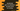# 3 JavaScript programs to get the first character of each words in a string### Introduction:

In this post, I will show you how to get the first character of each word in a string in JavaScript. We will write one program that will take one string as input from the user and prints out the first character of each word in that string as output. If our string is Hello World and Hello Universe, It will return the characters H,W,a,H,U.

This problem can be solved in multiple ways. The easiest way to solve this is by iterating through the characters of the string one by one. Or we can go with ES6 to do that in one line or we can use regex or regular expression.

In this post, I will show you all these three different ways to get the first character of each word in JavaScript.

### Method 1: Using a loop:

Using a loop, we can iterate through the characters of a string and get the first character of each word of that string. Below is the complete program:

``````function getFirstCharacters(str) {
// 1
if (str.length == 0) {
return [];
}

// 2
let result = [];
let blankFound = false;

// 3
if (str != " ") {
result.push(str);
}

// 4
for (ch of str) {
if (ch === " ") {
blankFound = true;
} else if (blankFound) {
blankFound = false;
result.push(ch);
}
}

// 5
return result;
}

const str1 = "Hello4 World65 123 !!";
const str2 = "123and 456 and 78-1";
const str3 = " Hello World    !!";

console.log(getFirstCharacters(str1));
console.log(getFirstCharacters(str2));
console.log(getFirstCharacters(str3));``````

#### Output:

This program will print the below output:

``````[ 'H', 'W', '1', '!' ]
[ '1', '4', 'a', '7' ]
[ 'H', 'W', '!' ]``````

Each array holds the first character of words in the string we provided.

#### Explanation:

The commented numbers in the program denote the step numbers below :

1. getFirstCharacters function is used to get the first characters of each word in a string. It takes one string as its parameter and returns one array holding the first character of each word in the string. If the provided string is empty, we are returning one empty array
2. result is an empty array used to hold the first characters. blankFound is a boolean value denotes if any blank character is found. If found, we will add the next character to the array.
3. In this step, we are checking if the first character of the string is space or not. If not, we are adding it to the array.
4. This is a for-of loop to iterate through the characters of the string one by one. For each character, we are checking if it is empty or not. If yes, we are assigning blankFound as true. On next iteration, if blankFound is true and if we are getting a non empty character, adding that to the array and reassigning blankFound to false.
5. Finally, that array result is returned.

## Method 2: Using ES6 map and split:

split is used to split one string to words. For example, for the string Hello World !!, if we call split(’ ‘) on this string, it will return one array holding Hello, World and !!.

We can call map to this array of words and get the first character of each word and put them in an array.

Below is the complete program:

``````function getFirstCharacters(str) {
let result = [];

str.split(' ').map(word => word.charAt(0) != '' ? result.push(word.charAt(0)) : '');

return result;
}

const str1 = "Hello4 World65 123 !!";
const str2 = "123and 456 and 78-1";
const str3 = " Hello World    !!";

console.log(getFirstCharacters(str1));
console.log(getFirstCharacters(str2));
console.log(getFirstCharacters(str3));``````

#### Output:

It will print :

``````[ 'H', 'W', '1', '!' ]
[ '1', '4', 'a', '7' ]
[ 'H', 'W', '!' ]``````

#### Explanation:

In this example, we are using only one line to solve it. It :

• splits the string in blank space
• map all words in the array of words
• check for each word, if the first character is empty or not. If it is not empty, push the character to the final result array. Else, do nothing.

That’s all.

## Method 3: Using regex:

Regex is bit difficult and we need to verify it on different strings to check if it works on all or not. I take this regex pattern from here and you can check with different patterns and different strings.

``````function getFirstCharacters(str) {
let result = [];

return str.match(/(?:\s|^)(\S)/g).join(',');

return result;
}

const str1 = "Hello4 World65 123 !!";
const str2 = "123and 456 and 78-1";
const str3 = " Hello World    !!";

console.log(getFirstCharacters(str1));
console.log(getFirstCharacters(str2));
console.log(getFirstCharacters(str3));``````

It will print:

``````H, W, 1, !
1, 4, a, 7
H, W, !``````